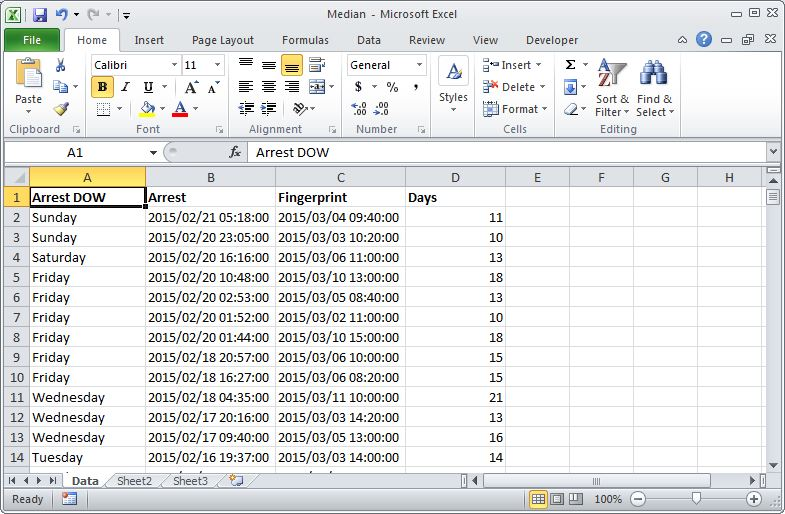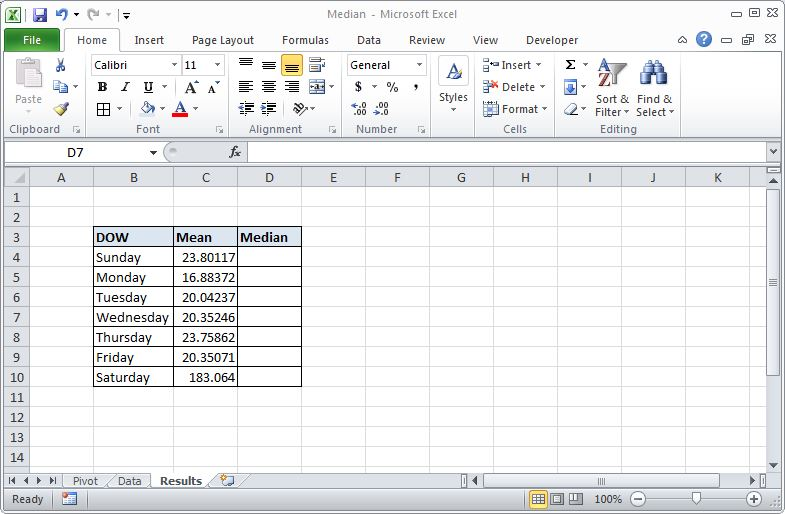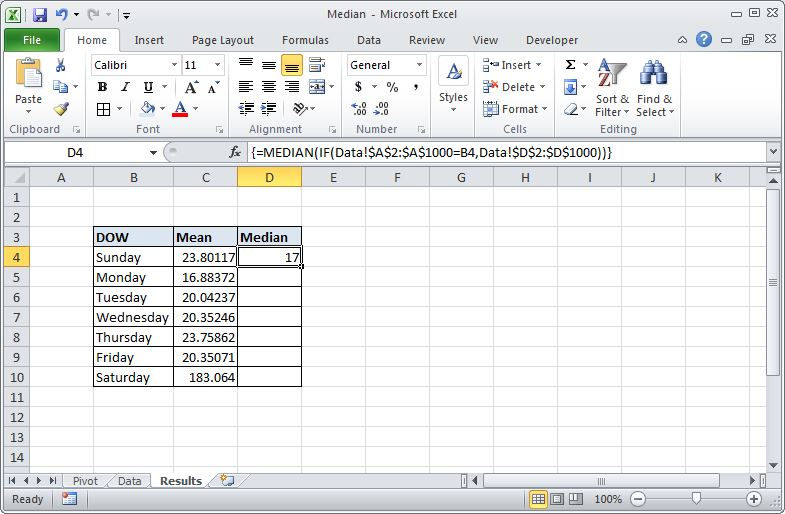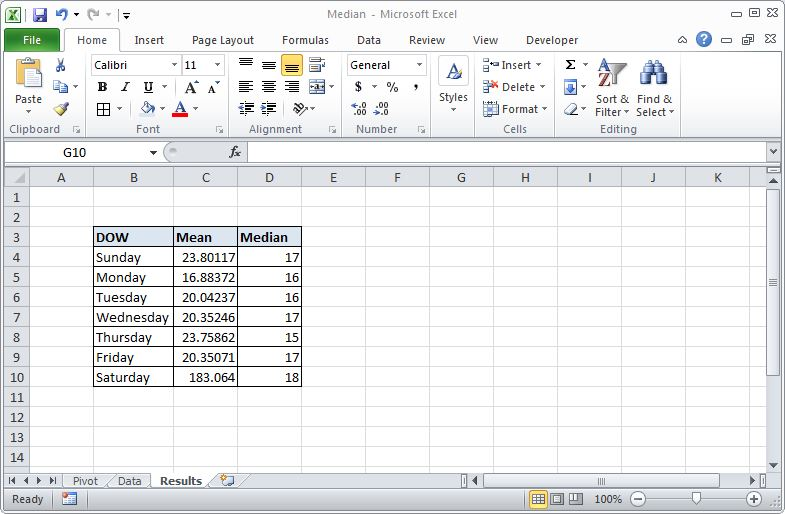### Medians and Excel Pivot Tables

One of the issues with pivot tables in Excel 2010 (and earlier versions) is that it isn’t possible to calculate median using the Value Field Settings. In this post I am going to first extol the virtues of the median (again) and then demonstrate a formula for calculating the median in a cross tabulation.

Medians Again?

Why do we care about the median and why can’t we just use the mean? The mean is the proper measure of central tendency when what we want to average is normally distributed. In those situations, adding everything up and dividing by the number of elements will give the value right at the peak of the distribution. However, when our distribution of values is heavily skewed the mean is less representative than the median. In those cases it makes more sense to take the middle value (i.e. the median) of all the values when they are ordered from smallest to largest.

The classic example of using the median is household income. There are a lot more small income households than large income households and those big households can really distort the mean. For example, let’s say that we have 7 households with incomes (in \$10,000s) of 1, 1.4, 1.8, 2, 2.1, 2.4 and 13.2. The mean of those numbers is 3.5 while the median is 2. Clearly 2 is a better representation of the centre of the data than the mean, which has been dragged higher by the one large income.

A similar issue occurs with any data set that is zero based.  For example, say you want to do an analysis to determine how long offenders wait between their arrest and when they are fingerprinted. Many services schedule identification appointments days or even weeks after the arrest and it might be interesting to see what the average time is between arrest and appointment. The shortest possible wait is 0 days, the fingerprinting is done on the day of the arrest, and in general one would expect a shorter time rather than a longer one. While there may be some 28-day waits we don’t want these outliers to unduly skew our average so we choose to take the median rather than the mean.

An Example

Let’s take this example further by considering a spreadsheet were we have 1000 rows of arrest times and fingerprint times and the duration in days between them.The easiest way to determine the median would be to use the formula =MEDIAN(D2:D1000) but that would only give us the median for the entire data set. When analysing our data with pivot tables we are often interested in cross tabulating based on a particular element of the data and in this example we want to see if average wait time is impacted by the day of the week of the arrest.

To begin the analysis we first insert a new pivot table and use it to determine average (by which Excel means mean) wait time for each day of the week.Have a look at these mean values, particularly Saturday. What is up with that? I’ll tell you: there are errors in the data set. Someone has keyed the dates in incorrectly and there are outliers that are horribly skewing the results. This is the other benefit of working with the median: medians resist the impact of outrageous outliers in a way that the mean doesn’t. We could go back in and do some data cleansing but let’s leave it for now so we can see how the median will handle it.

If you look at the list of options in the Value Field Settings you’ll see that Median is not an option. Since pivot tables don’t provide the option for determining the median we need to do some copying and pasting and use a formula to make it happen. Whenever I am investigating the median I first copy-and-paste-values the results of the mean pivot table to a new work sheet so that I can use the labels in my function calls. The new worksheet looks like this.To calculate the median for each of the days of the week it is necessary to pull only those rows of data in the Data worksheet for that match the particular day. Once I have those rows I use the MEDIAN function to calculate the specific median for that day of week. To do this I use the formula in cell D4 below:It is important to notice that the formula in the screen shot is surrounded by curly braces. You don’t type the braces in because Excel adds them when you create what’s known as an Array Formula. Array formulas are different than regular Excel formulas because they work with a whole series of data values rather than a single data value. To tell Excel that you intend a formula to be an array formula you must press CTRL SHIFT ENTER (all three keys at once, like CTRL ALT DEL) at the end, rather than just hitting ENTER. You’ll know that you did it right because Excel will add the curly braces.

In this particular array formula what we’re doing is using an IF formula to check every one of the Day of Week values in column A of the Data worksheet to see if it equals Sunday, or the value in B4. If the value does equal Sunday we return the wait time from column D for that row. The IF statement will check every row and return a range of values (all of the ones for Sunday) to the MEDIAN function which in turn calculates the median wait time for Sunday. The formula can then be filled down for each of the days just like you would with a regular formula.You can see in the screen grab that we have median values for each of the days of the week. Clearly the outlier that impacted Saturday has been handled by using the median but also note the other days (except Monday) now have significantly smaller values. This is further evidence that the median is the correct choice for this data as using the mean, even when the data is clean, would lead to erroneous overestimates.

Conclusion

The bad news is that Excel can’t calculate medians in a pivot table. The good news is that with a simple formula and the labels produced by the pivot table it is possible to calculate the median for different cross tabulations. It does require a little experimentation with array functions but this particular example is pretty tame and serves as a decent introduction to this very powerful technique.